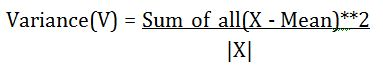# Python program to find the variance

Variance in python: Here, we are going to learn how to find the variance of given data set using python program?
Submitted by Anuj Singh, on June 30, 2019

While dealing with a large data, how many samples do we need to look at before we can have justified confidence in our answer? This depends on the variance of the dataset.

Variance tells us about the divergence and the inconsistency of the sample. So in this python article, we are going to build a function.

Mathematically we define it as:So the following function can be used while working on a program with big data which is very useful and help you a lot.

So here is the code:

```def variance(X):
mean = sum(X)/len(X)
tot = 0.0
for x in X:
tot = tot + (x - mean)**2

# main code
#  a simple data-set
sample = [1, 2, 3, 4, 5]
print("variance of the sample is: ", variance(sample))

sample = [1, 2, 3, -4, -5]
print("variance of the sample is: ", variance(sample))

sample = [10, -20, 30, -40, 50]
print("variance of the sample is: ", variance(sample))
```

Output:

```ariance of the sample is:  2.0
variance of the sample is:  10.64
variance of the sample is:  1064.0
```

Preparation